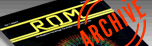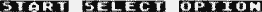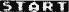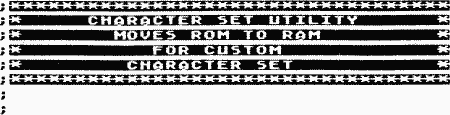`ROM ISSUE 9 — DECEMBER/JANUARY 1985 / PAGE 8`

 ACTION! CORNER by Kevin Greggain     Computer languages come and go with time because of many factors. One of these is speed. How fast a program will run is very important to someone who is writing a fast-action game. If it was written in BASIC, the game would be too slow. To take the big step and write the game in assembly language would take a long time with a lot of hard work. This is where ACTION! comes along. Although it is a high-level language, like BASIC, when compiled RUNS quickly like a low-level language.     The thing that makes this language so unique is how easy a BASIC programmer could switch over to using it. A complete BASIC program could be converted over to ACTION! and may run over 10 times as fast. In this article, I'm first giving you a program that will run a number of spectacular graphic demos. These demos, if written in BASIC, would be so slow they'd be boring, but in ACTION!, they are fast and colorful. Each demo has comments to make them easier to understand.     The second program is just a short one that moves the character set from ROM to RAM for use in your own custom character set. In the next issue a complete listing to a game written in ACTION! will be given. MODULE               ;WE'RE GOING TO D ECLARE SOME BYTE C4=704,         ;GLOBAL VARIABLES  FOR USE BY THE      C5=705,         ;PROCEDURE CALLS      C6=706,         ;C4-C12 ARE THE R EGISTERS FOR MODE 10      C7=707,      C8=708,      C9=709,      C10=710,      C11=711,      C12=712,      LM,RM,      BM,RO,      LOOP,C=E17,     ;C IS THE POINTER  VARIABLE USED FOR THE COLOR      ATT=77,         ;ATTRACT MODE AT 77 IS ALWAYS RESET TO 0      LOOP1,LOOP2,      CONSOLE=53279   ;THE CONSOLE KEYSPROC COL()           ;THIS ROUTINE SET S THE SEOUENTIAL BUT RANDOM COLORS TO DRAW WITH BYTE NUM NUM=RAND(14)*14 C4=0 C5=NUM CG=NUM+2 C7=NUM+4  ;SETS C OLORS TO COLOR BASE TO COLOR BASE+14 C8=NUM+6 C9=NUM+8 C10=NUM+10   ;SAME A 5 BASIC POKE C11=NUM+12 C12=NUM+14 RETURN PROC SCROLL()                  ;THIS I S THE ROUTINE TO PUT IT ALL TOGETHER O NCE IT'S DRAWN BYTE WSYNC=54282,              ;WAIT S YNC LOCATION      VCOUNT=54283,             ;VERTIC AL LINE COUNTER      CLOCK=20,                 ;RTC CL OCK AT 18,19,20 USED FOR TIMING      CLOCK2=19,                ;      CLOCK3=540,               ;JIFFY  COUNTER      C01=\$D012,                ;SCREEN   REGISTER FOR GRAPHICS 10      TEMP                      ;TEMPOR ARY STORAGE VARIABLE FOR LOCAL USE CLOCK2=0 CLOCK3=240            ;SET CL OCK TIMERS DO                             ;BEGIN LOOP      IF CLOCKS=0 THEN          ;COMPAR E 540 WITH 0 (CLOCK COUNTS BACKWARDS F ROM 240 AS ORIGINALLY SET)        TEMP=C12                ;STORE REGISTER INTO TEMP..SIMILAR TO PHA IN ASSEMBLY]        C12=C11                 ;THESE FOLLOWING MOVES SWAP THE COLOR REGISTE RS        C11=C10        C10=C9        C9=C8        C8=C7        C7=C6        C6=C5        C5=TEMP      CLOCKS=2                  ;RESET 540 TO 2 (2 60ths OF A SECOND)      FI      IF VCOUNT<=61 THEN        ;CHECK 54283 FOR <=61 (APP MID SCREEN)        WSYNC=1                 ;STORE AT NSYNC        C01=VCOUNT+CLOCK        ;STORE COLOR IN REGISTER \$D012                                ;IS CON DITION TRUE                                ;NO SO GO TO SECOND PART      ELSEIF VCOUNT>=62 THEN    ;BIGGER THAN MID SCREEN?       WSYNC=1                  ;STORE AGAIN       C01=VCOUNT-CLOCK         ;STORE COLOR -CLOCK FOR REVERSE SCROLLING EFF ECT      FI                        ;END OF CHECK UNTIL CLOCK2=10 OR CONSOLE=6   ;LOOK F OR CLOCK 19=10 OR START KEY PRESSED (C ONSOLE=6 =START) OD                             ;END OF SCROLL ROUTINE ATT=0                          ;TURN O FF ATTRACT IF ON RETURN                         ;ATTRAC T ENABLES AFTER LOCATION 77>=128 PROC DR()                      ;THE DR # PROCEDURES ARE USED BY THE MAIN ROUT INE TO DRAW THE IMAGES. LM=0 RM=79 BM=191 RO=0         ;WE SET  THE LEFT RIGHT BOTTOM AND TOP MARGINS  FOR USE WITHIN THE PROGRAM GRAPHICS(10) C4=0              ;SET UP   MODE FOR SCREEN AND SET SCREEN COLOR TO 0 (BLACK) COL ()                         ;CALLS BACK TO THE COL() PROCEDURE WHICH SETS THE COLOR REGISTERS DO       COLOR=C       PLOT (LM,RO)       DRAWTO(RM,RO)       DRAWTO(RM,BM)       DRAWTO(LM,BM)       DRAWTO(LM,RO)       C==+1 IF C>6 THEN C=1    ;THIS C HECKS FOR C AND RESETS IF COLOR > 8 TO   1       FI       LM==+1 PM==-1 BM==-1 RO==+1 UNTIL LM=79 OD RETURN PROC DR2() LM=0 RM=79 BM=191 RO=0 GRAPHICS(10) COL C1 DO FOR LOOP=LM TO RM DO   COLOR=C   PLOTCLOOP,RO) C==+1   IF C>9 THEN C=1 FI   OD FOR LOOP=RO TO BM DO   COLOR=C   PLOT(RM,LOOP) C==-1   IF C<1 THEN C=9 FI   OD LOOP=RM   DO   COLOR=C   PLOT(LOOP,BM) C=C+1   IF C>9 THEN C=1 FI   LOOP==-1   UNTIL LOOP=LM   OD LOOP=BM   DO   COLOR=C   PLOTCLM,LOOP7 C=C-1   IF C<1 THEN C=9 FM   LOOP==-1   UNTIL LOOP=RO   OD LM==+1 RM==-1 BM==-1 RO==+1 UNTIL LM=40 OD LM=39 RO=95 BM=95 RM=39 DO          COLOR=C          PLOT (LM, RO) DRAWTO(RM,RO)          DRAWTO(RM,BM) DRAWTO(LM,BM)          DRAWTO(LM,RO)          C==-1 ZF C<1 THEN C=8 FI          LM==-1 RM4==+1 RO==-1 BM==+1          UNTIL LM=10 OD RETURN PROC DR3() BYTE X,Y GRAPHICS(10) COL () FOR LOOP=0 TO 100 DO        COLOR=RAND(9)+1        X=RAND(79)        Y=RAND(100)        PLOT(X,Y)        OD LM=0 RM=79 BM=191 RO=10 DO        COLOR=C        PLOT(LM,RO) DRAWTO(LM,BM)        DRAWTO(RM,BM) DRAWTO(RM,RO)        C==-1 IF C<1 THEN C=8 FI        LM==+1 RM==-1 BM==-1 R0==+3 UNTIL LM=37 OD RETURN PROC DR4() GRAPHICS(10) COL() FOR LOOP1=30 TO 50 STEP 2      DO      COLOR=C      C==+1 IF C>8 THEN C=1 FI            FOR LOOP2=LOOP1 TO LOOP1+2            DO            PLOT(5,LOOP2) DRAWTO(74,LOO P2)            OD      OD FOR LOOP1=150 TO 189      DO      COLOR=C      C==+1 IF C>8 THEN C=1 FI      PLOT(0,LOOP1) DRAWTO(79,LOOP1)      OD FOR LOOP1= 5 TO 70 STEP 9      DO      C=1         FOR LOOP2 =LOOP1 TO LOOP1+6         DO         COLOR=C         C==+1 ZF C>8 THEN C=1 FI         PLOT(LOOP2,149) DRAWTO(LOOP2,5 3)         OD      OD LOOP1=39 LOOP2=39 RO=0      DO      COLOR=C      PLOT(LOOP1,R0) DRAWTO(LOOP2,R0)      R0==+1 LOOP1==-1 LOOP2==+1      C==+1 IF C>8 THEN C=1 FI      UNTIL RO=30      OD C=0 FOR LOOP1 = 35 TO 45      DO      COLOR=C      PLOT(20,LOOP1) DRAWTO(59,LOOP1)      OD RETURN PROC DR5() GRAPHICS(10) COL() LM=0 RM=79 BM=95 RO=0 DO        COLOR=C        PLOT(LM,R0) DRAWTO(RM,R0)        DRAWTO(RM,BM) DRAWTO(LM,BM)        DRAWTO(LM,RO)        PLOT(LM,95+RO) DRAWTO(RM,95+RO)        DRAWTO(RM,95+BM) DRAWTO(LM,95+8 M)        DRAWTO(UM, 95+RO)        C==+1 IF C>8 THEN C=1 FI        LM==+1 RM==-1 BM==-1 RO==+1        UNTIL LM=69 OD RETURN PROC INTRO()         ;I WILL ADD SOME INSTRUCTIONS HERE GRAPHICS(10)        ;GRAPHICS 24+16 (W ITHOUT TEXT WINDOW) POSITION(1,0) PRINTDE(6,"useto quick")  ;PR INTDE(6,"TEXT") IS THE METHOD POSITION(1,2)                      ;TH AT ACTION USES FOR THE EQUIVALENT OF B ASIC'S PRINTDE(6,"advance pictures or ")  ;PR INT#6;;"TEXT" POSITZON(1,4) PRINTDE(6,"Wait and they Will") POSITION(0,6) PRINTDE(6,"change after a short") POSITION(2,8) PRINTDE(6,"period of time") POSITION(0,10) PRINTDE(6,"pressto go on") DO                                 ;BE GIN LOOP UNTIL CONSOLE =6 OD                ;AN D WAIT TILL START IS PRESSED RETURN PROC MAIN()       ;THIS ZS THE MAIN RO UTINE WHICH CALLS BACK TO THE DRAW ROU TINES.                   ;NOTICE HOW NICE YOU  CAN FORMAT THE TEXT TO SUIT YOUR READ ING NEEDS INTRO () DO DR1() SCROLL()    ;THIS CALLS THE DR1 ROUTINE WHICH CALLS COL() THEN SCROLL(   )ETC. DR2() SCROLL()    ;AND 2 DR3() SCROLL()    ; DR4() SCROLL()    ; DR5() SCROLL()    ;AND DOWN TO 5 OD                ;CONTINUE FOREVER RETURN ;YOU CAN ADD YOUR OWN DRAWING ROUTINES  TO THE SYSTEM BY ;JUST LABELING THEM DR# WHERE NUMBER I S THE ROUTINE TO USE ;REMEMBER TO ADD THE ROUTINE CALL INTO  THE MAIN() ROUTINE AND CALL THE SCROL L() ROUTINE TO GET ;THE RAINBOWING EFFECT AFTER YOU ARE F INZSHED.. ;THE TIMER VALUES IN SCROLL() CAN BE I NCREASED TO SUIT YOUR VIEWING TIMES. PROC CSET()BYTE CHBASE=756,              ;POINTE R FOR CHARACTER BASE      RAMTOP=106,              ;TOP OF  FREE RAM (USUALLY 40960)      WSYNC=54282,             ;WAIT S YNC      VCOUNT=54283,            ;VERTIC AL LINE COUNTER      CO1=\$D01A,               ;HARDWA RE REGISTER FOR SCREEN (GR.18)      CO2=\$D016.               ;HARDWA RE REGISTER FOR 708      CLOCK=20                 ;RTC AT  18,19,20 CARD ROMSET,                  ;VARIAB LE FOR STANDARD SET      LOOP                     ;FOR LO ADING IN CHARACTER SET BYTE ARRAY NEWSET             ;VARIAB LE FOR NEW SET RAMTOP==-4 GRAPHICS(18)       ;4 PAGE S-1024 BYTES                               ;GRAPHI CS 0 RE INITS POINTER NEWSET=RAMTOP*256             ;SET UP  NEW START FOR CHARACTER DATA ROMSET=CHBASE*256             ;ESTABL ISH OLD ROM CHARACTER SET MOVEBLOCK(NEWSET,ROMSET,1024) ;ZAPP.. COPY OLD ROM DATA TO NEW LOCATION CHBASE=NEWSET/256             ;POINT TO MY NEW SET CLOSE(1) OPEN(1,"D1:FANCY",4,0);OPEN C USTOM CHARACTER SET DATA FILE FOR LOOP=0 TO 1024   DO       NEWSET(LOOP)=GETD(1)      ;GET AN D STORE BYTE IN NEW CHARACTER SET LOCA TION   OD       CLOSE (1)